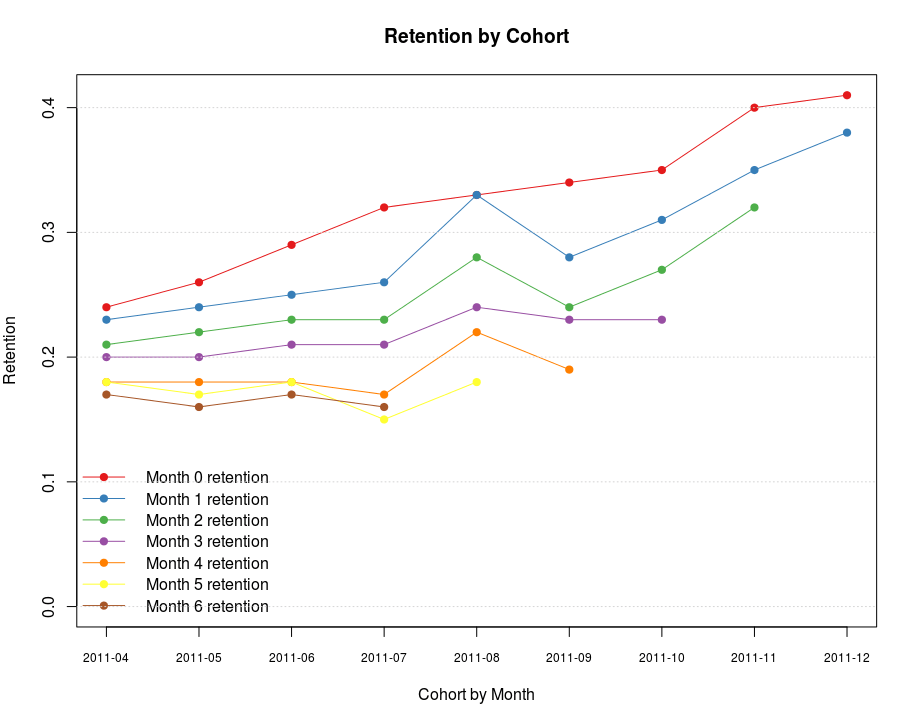Want to share your content on R-bloggers? click here if you have a blog, or here if you don't.

Cohort analysis is super important if you want to know if your service is in fact a leaky bucket despite nice growth of absolute numbers. There’s a good write up on that subject “Cohorts, Retention, Churn, ARPU” by Matt Johnson.

So how to do it using R and how to visualize it. Inspired by examples described in “Retention, Cohorts, and Visualizations” I came up with the following solution.

First, get the data in a suitable format, like this:

```cohort  signed_up  active_m0  active_m1  active_m2
2011-10 12345      10432      8765       6754
2011-11 12345      10432      8765       6754
2011-12 12345      10432      8765       6754```

Cohort here is in “YYYY-MM” format, signed_up is the number of users who have created accounts in the given month, active_m0 – number of users who have been active in the same month as they registered, active_m1 – number of users who have been active in the following month, and so forth. For newest cohorts you’ll be getting zeroes in some of active_mN columns, since there’s no data on them yet. This is taken into account in processing scripts.

```require(plyr)

con = gzcon(url('http://www.systematicportfolio.com/sit.gz', 'rb'))
source(con)
close(con)

cohorts
# Let's convert absolute values to percentages (% of the registered users remaining active)
cohort_p as.numeric(df\$active_m0/df\$signed_up), as.numeric(df\$active_m1/df\$signed_up), as.numeric(df\$active_m2/df\$signed_up),
as.numeric(df\$active_m3/df\$signed_up), as.numeric(df\$active_m4/df\$signed_up), as.numeric(df\$active_m5/df\$signed_up),
as.numeric(df\$active_m6/df\$signed_up), as.numeric(df\$active_m7/df\$signed_up), as.numeric(df\$active_m8/df\$signed_up) ))

# Create a matrix
temp = as.matrix(cohort_p[,3:(length(cohort_p[1,])-1)])
colnames(temp) = paste('Month', 0:(length(temp[1,])-1), sep=' ')
rownames(temp) = as.vector(cohort_p\$V1)

# Drop 0 values and format data
temp[] = plota.format(100 * as.numeric(temp), 0, '', '%')
temp[temp == " 0%"] # Plot cohort analysis table
plot.table(temp, smain='Cohort(users)', highlight = TRUE, colorbar = TRUE)
```

This code produces nice visualizations of the cohort analysis as a table:I used articles “Visualizing Tables with plot.table“ and “Response to Flowingdata Challenge: Graphing obesity trends” as an inspiration for this R code.

If you want to get nice colours as in the example above, you’ll need to adjust rainbow interval for plot.table. I managed to do it by editing functions code directly from R environment:

```plot.table.helper.color <- edit(plot.table.helper.color)

function
(
temp # matrix to plot
){
# convert temp to numerical matrix
temp = matrix(as.double(gsub('[%,\$]', '', temp)), nrow(temp), ncol(temp))

highlight = as.vector(temp)
cols = rep(NA, len(highlight))
ncols = len(highlight[!is.na(highlight)])
cols[1:ncols] = rainbow(ncols, start = 0, end = 0.3)

o = sort.list(highlight, na.last = TRUE, decreasing = FALSE)
o1 = sort.list(o, na.last = TRUE, decreasing = FALSE)
highlight = matrix(cols[o1], nrow = nrow(temp))
highlight[is.na(temp)] = NA
return(highlight)
}
```

Adjust interval in line 11 to 0.5, 0.6 to get shades of blue.
plot.table.helper.colorbar <- edit(plot.table.helper.colorbar)

```function
(
plot.matrix # matrix to plot
)
{
nr = nrow(plot.matrix) + 1
nc = ncol(plot.matrix) + 1

c = nc
r1 = 1
r2 = nr

rect((2*(c - 1) + .5), -(r1 - .5), (2*c + .5), -(r2 + .5), col='white', border='white')
rect((2*(c - 1) + .5), -(r1 - .5), (2*(c - 1) + .5), -(r2 + .5), col='black', border='black')

y1= c( -(r2) : -(r1) )

graphics::image(x = c( (2*(c - 1) + 1.5) : (2*c + 0.5) ),
y = y1,
z = t(matrix( y1 , ncol = 1)),
col = t(matrix( rainbow(len( y1 ), start = 0.5, end = 0.6) , ncol = 1)),
}
```

Adjust interval in line 21 to 0.5, 0.6 to get shades of blue.

Now if you want to draw the cycle-like graph:

```# make matrix shorter for the graph (limit to 0-6 months)
temp = as.matrix(cohort_p[,3:(length(cohort_p[1,])-1)])
temp temp[temp == "0"]
library(RColorBrewer)
colnames(temp) = paste('Month', 0:(length(temp[1,])-1), 'retention', sep=' ')
palplot(temp[,1],pch=19,xaxt="n",col=pal,type="o",ylim=c(0,as.numeric(max(temp[,-2],na.rm=T))),xlab="Cohort by Month",ylab="Retention",main="Retention by Cohort")

for(i in 2:length(colnames(temp))) {
points(temp[,i],pch=19,xaxt="n",col=pal[i])
lines(temp[,i],pch=19,xaxt="n",col=pal[i])
}

axis(1,at=1:length(cohort_p\$cohort),labels=as.vector(cohort_p\$cohort),cex.axis=0.75)
legend("bottomleft",legend=colnames(temp),col=pal,lty=1,pch=19,bty="n")
abline(h=(seq(0,1,0.1)), col="lightgray", lty="dotted")
```

This code produces nice visualizations of the cohort analysis as multicolour cycle graph: